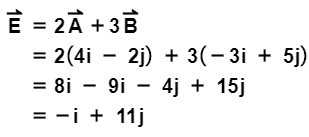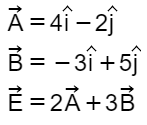# Problem: LetWhat is the magnitude of vector E⃗?

###### FREE Expert Solution

2-D vector magnitude:

$\overline{)\mathbf{|}\stackrel{\mathbf{⇀}}{\mathbf{r}}\mathbf{|}{\mathbf{=}}\sqrt{{\mathbf{x}}^{\mathbf{2}}\mathbf{+}{\mathbf{y}}^{\mathbf{2}}}}$96% (400 ratings)###### Problem Details

LetWhat is the magnitude of vector E⃗?